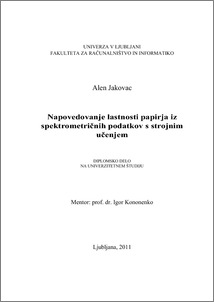# The prediction of paper properties from spectrometric data with machine learning

Alen Jakovac (2011) The prediction of paper properties from spectrometric data with machine learning. EngD thesis.Preview
PDF

## Abstract

In this thesis we present a solution for the problem of predicting the chemical and physical properties of paper from spectrometric data. We used a data set that consists of over 1000 samples of paper. For each sample 15 chemical and physical properties and its near-infrared spectra were measured. We used the following machine learning methods to predict the properties of paper: linear regression, pace regression, a nearest neighbor-based model, regression trees, a support vector machine, principal component regression, partial least squares regression, a multi-layer perceptron, and a radial basis function network. The prediction task turned out to be linear. Therefore, linear regression, principal component regression, and partial least squares regression gave the best results. Many outside factors affect the spectra and cause different types of interference. We used the following spectra preprocessing methods to remove the interference and improve the predictions: absorbance transformation, Kubelka-Munk transformation, multiplicative scatter correction, standard normal variate transformation, spectra derivation and orthogonal signal correction. We also investigated how preprocessing affects the machine learning methods. The results show that most preprocessing methods improve the models' predictions. The standard normal variate transformation and multiplicative scatter correction gave the best results. We tried to further improve the predictions with calibration. However, calibration did not improve the predictions.

Item Type: Thesis (EngD thesis)
Keywords: NIR spectroscopy, chemical and physical properties of paper, preprocessing NIR spectra, machine learning, calibration
Number of Pages: 86
Language of Content: Slovenian
Mentor / Comentors:
Name and SurnameIDFunction
prof. dr. Igor Kononenko237Mentor